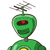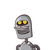# How many 5 – digit numbers divisible by 4 can be formed using the digits 5,6, 7, 8, and 9 such that there is no repetition o

How many 5 – digit numbers divisible by 4 can be formed using the digits 5,
6, 7, 8, and 9 such that there is no repetition of digits?
A)
30
B)
21
C)
24
D)
18​

### 2 thoughts on “How many 5 – digit numbers divisible by 4 can be formed using the digits 5,<br />6, 7, 8, and 9 such that there is no repetition o”

1.24

Step-by-step explanation:

2.important number is only last two digit to find ita divide by 4

you note only even number

• first check if last digit is 6
• second check last digit 8

56,76 two numbers is divided by 4 so first 3 digit arrangement = 3!×2 =12

68 is only number which divide by 4 =3!×1 =6